Learning never stops. When SAS had to change this year’s SAS Global Forum (SGF) to a virtual event, everyone was disappointed. I am, however, super excited about all of the papers and stream of video releases over the last month (and I encourage you to register for the upcoming live event in June). For now, I made a pact with myself to read or watch one piece of SGF related material per day. While I haven’t hit my goal 100%, I sure have learned a lot from all the reading and viewing. One particular paper, Using Jupyter to Boost Your Data Science Workflow, and its accompanying video by Hunter Glanz caught my eye this week. This post elaborates on one piece of his material: how to save Jupyter notebooks in other file formats.

# Hunter’s story

Hunter is a professor who teaches multiple classes using SAS® University Edition, which comes equipped with an integrated Jupyter notebook. His focus is on SAS programming and he requires his students to create notebooks to complete assignments; however he wants to see the results of their work, not to run their raw code. The notebooks include text, code, images, reports, etc. Let's explore how the students can transform their navitve notebooks into other, more consumable formats. We'll also discuss other use cases in which SAS users may want to create a copy of their work from a notebook, to say a .pdf, .html, or .py file, just to name a few.

# What you’ll find here and what you won’t

This post will not cover how to use Jupyter notebooks with SAS or other languages. There is a multitude of other resources, starting with Hunter’s work, to explore those topics. This post will cover how to produce other file formats in SAS, Python, and R. I’ll outline multiple methods including a point-and-click method, how to write inline code directly in the notebook, and finally using the command line.

Many of the processes discussed below are language agnostic. When there are distinct differences, I’ll make a note.

# A LITTLE about Jupyter notebooks

A Jupyter notebook is a web application allowing clients to run commands, view responses, include images, and write inline text all in one concourse. The all-encompassing notebook supports users to telling complete story without having to use multiple apps. Jupyter notebooks were originally created for the Python language, and are now available for many other programming languages. JupyterLab, the notebooks’ cousin, is a later, more sophisticated version, but for this writing, we’ll focus on the notebook. The functionality in this use case is similar.

Where do we start? First, we need to install the notebook, if you're not working in a SAS University Edition.

## Install Anaconda

The easiest way to get started with the Jupyter Notebook App is by installing Anaconda (this will also install JupyterLab). Anaconda is an open source distribution tool for the management and deployment of scientific computing. Out-of-the-box, the notebook from the Anaconda install includes the Python kernel. For use with other languages, you need to install additional kernels.

In this post, we’ll focus on Python, R, and SAS. The Python kernel is readily available after the Anaconda install. For the R language, follow the instructions on the GitHub R kernel repository. I also found the instructions on How to Install R in Jupyter with IRKernel in 3 Steps quite straight forward and useful. Further, here are the official install instructions for the SAS kernel and a supporting SAS Community Library article.

With the additional kernels are in place, you should see all available languages when creating a new notebook as pictured below.

# File conversion methods

Now we’re ready to dive into the export process. Let’s look at three approaches in detail.

Once you’ve opened your notebook and run the code, select File-> Download As (appears as Export Notebook As… in JupyterLab).

### HTML format output

Notice the list of options, some more familiar than others. Select the HTML option and Jupyter converts your entire notebook: text, commands, figures, images, etc, into a file with a .html extension. Opening the resulting file would display in a browser as expected. See the images below for a comparison of the .ipynb and .html files.

### SAS (aka script) format output

Using the Save As-> SAS option renders a .sas file and is depicted in Enterprise Guide below. Note: when using a different kernel, say Python or R, you have the option to save in that language specific script format.

One thing to note here is only the code appears in the output file. The markdown code, figures, etc., from the original notebook, are not display options in EG, so they are removed.

### PDF format output

There is one (two actually) special case(s) I need to mention. If you want to create a PDF (or LaTeX, which is used to create pdf files) output of your notebook, you need additional software. For converting to PDF, Jupyter uses the TeX document preparation ecosystem. If you attempt to download without TeX, the conversion fails, and you get a message to download TeX. Depending on your OS the TeX software will have a different name but will include TeX in the name. You may also, in certain instances, need Pandoc for certain formats. I suggest installing both to be safe. Install TeX from its dowload site. And do the same for Pandoc.

Once I’ve completed creating the files, the new files appear in my File Explorer.

### Cheaters may never win, but they can create a PDF quickly

Well, now that we’ve covered how to properly convert and download a .pdf file, there may be an easier way. While in the notebook, press the Crtl + P keys. In the Print window, select the Save to PDF option, choose a file destination and save. It works, but I felt less accomplished afterward. Your choice.

## Inline code option

Point-and-click is a perfectly valid option, but let’s say you want to introduce automation into your world. The jupyter nbconvert command provides the capability to transform the current notebook into any format mentioned earlier. All you must do is pass the command with a couple of parameters in the notebook.

In Python, the nbconvert command is part of the os library. The following lines are representative of the general structure.

```import os os.system("jupyter nbconvert myNotebook.ipynb --to html")```

### An example with Python

The example below is from a Python notebook. The "0" out code represents success.

### An example with SAS

As you see with the Python example, the code is just that: Python. Generally, you cannot run Python code in a Jupyter notebook running the SAS kernel. Luckily we have Jupyter magics, which allow us to write and run Python code inside a SAS kernel. The magics are a two-way street and you can also run SAS code inside a Python shell. See the SASPy documentation for more information.

The code below is from a SAS notebook, but is running Python code (triggered by the %%python magic).

The EmployeeChurnSASCode.pdf file is created in same directory as the original notebook file:

### An example with R

Things are fairly straight forward in an R notebook. However, you must install and load the nbconvert package.

The first line installs the package, the second line loads the package, and the third actually does the conversion. Double-check your paths if you run into trouble.

## The command line

The last method we look at is the command line. This option is the same regardless of the language with which you’re working. The possibilities are endless for this option. You could include it in a script, use it in code to run and display in a web app, or create the file and email it to a colleague. The examples below were all run on a Windows OS machine using the Anaconda command prompt.

### An example with a SAS notebook

Convert sasNotebook.ipynb to a SAS file.

```>> ls -la |grep sasNotebook -rw-r--r-- 1 jofurb 1049089 448185 May 29 14:34 sasNotebook.ipynb   >> jupyter nbconvert --to script sasNotebook.ipynb [NbConvertApp] Converting notebook sasNotebook.ipynb to script [NbConvertApp] Writing 351 bytes to sasNotebook.sas   >> ls -la |grep sasNotebook -rw-r--r-- 1 jofurb 1049089 448185 May 29 14:34 sasNotebook.ipynb -rw-r--r-- 1 jofurb 1049089 369 May 29 14:57 sasNotebook.sas```

### An example with a Python notebook

Convert 1_load_data.ipynb to a PDF file

```>> ls -la |grep 1_load -rw-r--r-- 1 jofurb 1049089 6004 May 29 07:37 1_load_data.ipynb   >> jupyter nbconvert 1_load_data.ipynb --to pdf [NbConvertApp] Converting notebook 1_load_data.ipynb to pdf [NbConvertApp] Writing 27341 bytes to .\notebook.tex [NbConvertApp] Building PDF [NbConvertApp] Running xelatex 3 times: ['xelatex', '.\\notebook.tex', '-quiet'] [NbConvertApp] Running bibtex 1 time: ['bibtex', '.\\notebook'] [NbConvertApp] WARNING | b had problems, most likely because there were no citations [NbConvertApp] PDF successfully created [NbConvertApp] Writing 32957 bytes to 1_load_data.pdf   >> ls -la |grep 1_load -rw-r--r-- 1 jofurb 1049089 6004 May 29 07:37 1_load_data.ipynb -rw-r--r-- 1 jofurb 1049089 32957 May 29 15:23 1_load_data.pdf```

### An example with an R notebook

Convert HR_R.ipynb to an R file.

```>> ls -la | grep HR -rw-r--r-- 1 jofurb 1049089 5253 Nov 19 2019 HR_R.ipynb   >> jupyter nbconvert HR_R.ipynb --to script [NbConvertApp] Converting notebook HR_R.ipynb to script [NbConvertApp] Writing 981 bytes to HR_R.r   >> ls -la | grep HR -rw-r--r-- 1 jofurb 1049089 5253 Nov 19 2019 HR_R.ipynb -rw-r--r-- 1 jofurb 1049089 1021 May 29 15:44 HR_R.r```

# Wrapping things up

Whether you’re a student of Hunter’s, an analyst creating a report, or a data scientist monitoring data streaming models, you may have the need/requirement to transform you work from Jupyter notebook to a more consumable asset. Regardless of the language of your notebook, you have multiple choices for saving your work including menu options, inline code, and from the command line. This is a great way to show off your creation in a very consumable mode.

How to save Jupyter notebooks in assorted formats was published on SAS Users.Learning never stops. When SAS had to change this year’s SAS Global Forum (SGF) to a virtual event, everyone was disappointed. I am, however, super excited about all of the papers and stream of video releases over the last month (and I encourage you to register for the upcoming live event in June). For now, I made a pact with myself to read or watch one piece of SGF related material per day. While I haven’t hit my goal 100%, I sure have learned a lot from all the reading and viewing. One particular paper, Using Jupyter to Boost Your Data Science Workflow, and its accompanying video by Hunter Glanz caught my eye this week. This post elaborates on one piece of his material: how to save Jupyter notebooks in other file formats.

# Hunter’s story

Hunter is a professor who teaches multiple classes using SAS® University Edition, which comes equipped with an integrated Jupyter notebook. His focus is on SAS programming and he requires his students to create notebooks to complete assignments; however he wants to see the results of their work, not to run their raw code. The notebooks include text, code, images, reports, etc. Let's explore how the students can transform their navitve notebooks into other, more consumable formats. We'll also discuss other use cases in which SAS users may want to create a copy of their work from a notebook, to say a .pdf, .html, or .py file, just to name a few.

# What you’ll find here and what you won’t

This post will not cover how to use Jupyter notebooks with SAS or other languages. There is a multitude of other resources, starting with Hunter’s work, to explore those topics. This post will cover how to produce other file formats in SAS, Python, and R. I’ll outline multiple methods including a point-and-click method, how to write inline code directly in the notebook, and finally using the command line.

Many of the processes discussed below are language agnostic. When there are distinct differences, I’ll make a note.

# A LITTLE about Jupyter notebooks

A Jupyter notebook is a web application allowing clients to run commands, view responses, include images, and write inline text all in one concourse. The all-encompassing notebook supports users to telling complete story without having to use multiple apps. Jupyter notebooks were originally created for the Python language, and are now available for many other programming languages. JupyterLab, the notebooks’ cousin, is a later, more sophisticated version, but for this writing, we’ll focus on the notebook. The functionality in this use case is similar.

Where do we start? First, we need to install the notebook, if you're not working in a SAS University Edition.

## Install Anaconda

The easiest way to get started with the Jupyter Notebook App is by installing Anaconda (this will also install JupyterLab). Anaconda is an open source distribution tool for the management and deployment of scientific computing. Out-of-the-box, the notebook from the Anaconda install includes the Python kernel. For use with other languages, you need to install additional kernels.

In this post, we’ll focus on Python, R, and SAS. The Python kernel is readily available after the Anaconda install. For the R language, follow the instructions on the GitHub R kernel repository. I also found the instructions on How to Install R in Jupyter with IRKernel in 3 Steps quite straight forward and useful. Further, here are the official install instructions for the SAS kernel and a supporting SAS Community Library article.

With the additional kernels are in place, you should see all available languages when creating a new notebook as pictured below.

# File conversion methods

Now we’re ready to dive into the export process. Let’s look at three approaches in detail.

Once you’ve opened your notebook and run the code, select File-> Download As (appears as Export Notebook As… in JupyterLab).

### HTML format output

Notice the list of options, some more familiar than others. Select the HTML option and Jupyter converts your entire notebook: text, commands, figures, images, etc, into a file with a .html extension. Opening the resulting file would display in a browser as expected. See the images below for a comparison of the .ipynb and .html files.

### SAS (aka script) format output

Using the Save As-> SAS option renders a .sas file and is depicted in Enterprise Guide below. Note: when using a different kernel, say Python or R, you have the option to save in that language specific script format.

One thing to note here is only the code appears in the output file. The markdown code, figures, etc., from the original notebook, are not display options in EG, so they are removed.

### PDF format output

There is one (two actually) special case(s) I need to mention. If you want to create a PDF (or LaTeX, which is used to create pdf files) output of your notebook, you need additional software. For converting to PDF, Jupyter uses the TeX document preparation ecosystem. If you attempt to download without TeX, the conversion fails, and you get a message to download TeX. Depending on your OS the TeX software will have a different name but will include TeX in the name. You may also, in certain instances, need Pandoc for certain formats. I suggest installing both to be safe. Install TeX from its dowload site. And do the same for Pandoc.

Once I’ve completed creating the files, the new files appear in my File Explorer.

### Cheaters may never win, but they can create a PDF quickly

Well, now that we’ve covered how to properly convert and download a .pdf file, there may be an easier way. While in the notebook, press the Crtl + P keys. In the Print window, select the Save to PDF option, choose a file destination and save. It works, but I felt less accomplished afterward. Your choice.

## Inline code option

Point-and-click is a perfectly valid option, but let’s say you want to introduce automation into your world. The jupyter nbconvert command provides the capability to transform the current notebook into any format mentioned earlier. All you must do is pass the command with a couple of parameters in the notebook.

In Python, the nbconvert command is part of the os library. The following lines are representative of the general structure.

```import os os.system("jupyter nbconvert myNotebook.ipynb --to html")```

### An example with Python

The example below is from a Python notebook. The "0" out code represents success.

### An example with SAS

As you see with the Python example, the code is just that: Python. Generally, you cannot run Python code in a Jupyter notebook running the SAS kernel. Luckily we have Jupyter magics, which allow us to write and run Python code inside a SAS kernel. The magics are a two-way street and you can also run SAS code inside a Python shell. See the SASPy documentation for more information.

The code below is from a SAS notebook, but is running Python code (triggered by the %%python magic).

The EmployeeChurnSASCode.pdf file is created in same directory as the original notebook file:

### An example with R

Things are fairly straight forward in an R notebook. However, you must install and load the nbconvert package.

The first line installs the package, the second line loads the package, and the third actually does the conversion. Double-check your paths if you run into trouble.

## The command line

The last method we look at is the command line. This option is the same regardless of the language with which you’re working. The possibilities are endless for this option. You could include it in a script, use it in code to run and display in a web app, or create the file and email it to a colleague. The examples below were all run on a Windows OS machine using the Anaconda command prompt.

### An example with a SAS notebook

Convert sasNotebook.ipynb to a SAS file.

```>> ls -la |grep sasNotebook -rw-r--r-- 1 jofurb 1049089 448185 May 29 14:34 sasNotebook.ipynb   >> jupyter nbconvert --to script sasNotebook.ipynb [NbConvertApp] Converting notebook sasNotebook.ipynb to script [NbConvertApp] Writing 351 bytes to sasNotebook.sas   >> ls -la |grep sasNotebook -rw-r--r-- 1 jofurb 1049089 448185 May 29 14:34 sasNotebook.ipynb -rw-r--r-- 1 jofurb 1049089 369 May 29 14:57 sasNotebook.sas```

### An example with a Python notebook

Convert 1_load_data.ipynb to a PDF file

```>> ls -la |grep 1_load -rw-r--r-- 1 jofurb 1049089 6004 May 29 07:37 1_load_data.ipynb   >> jupyter nbconvert 1_load_data.ipynb --to pdf [NbConvertApp] Converting notebook 1_load_data.ipynb to pdf [NbConvertApp] Writing 27341 bytes to .\notebook.tex [NbConvertApp] Building PDF [NbConvertApp] Running xelatex 3 times: ['xelatex', '.\\notebook.tex', '-quiet'] [NbConvertApp] Running bibtex 1 time: ['bibtex', '.\\notebook'] [NbConvertApp] WARNING | b had problems, most likely because there were no citations [NbConvertApp] PDF successfully created [NbConvertApp] Writing 32957 bytes to 1_load_data.pdf   >> ls -la |grep 1_load -rw-r--r-- 1 jofurb 1049089 6004 May 29 07:37 1_load_data.ipynb -rw-r--r-- 1 jofurb 1049089 32957 May 29 15:23 1_load_data.pdf```

### An example with an R notebook

Convert HR_R.ipynb to an R file.

```>> ls -la | grep HR -rw-r--r-- 1 jofurb 1049089 5253 Nov 19 2019 HR_R.ipynb   >> jupyter nbconvert HR_R.ipynb --to script [NbConvertApp] Converting notebook HR_R.ipynb to script [NbConvertApp] Writing 981 bytes to HR_R.r   >> ls -la | grep HR -rw-r--r-- 1 jofurb 1049089 5253 Nov 19 2019 HR_R.ipynb -rw-r--r-- 1 jofurb 1049089 1021 May 29 15:44 HR_R.r```

# Wrapping things up

Whether you’re a student of Hunter’s, an analyst creating a report, or a data scientist monitoring data streaming models, you may have the need/requirement to transform you work from Jupyter notebook to a more consumable asset. Regardless of the language of your notebook, you have multiple choices for saving your work including menu options, inline code, and from the command line. This is a great way to show off your creation in a very consumable mode.

How to save Jupyter notebooks in assorted formats was published on SAS Users.I recently read an article that describes ways to compute confidence intervals for the difference in a percentile between two groups. In Eaton, Moore, and MacKenzie (2019), the authors describe a problem in hydrology. The data are the sizes of pebbles (grains) in rivers at two different sites. The authors ask whether the p_th percentile of size is significantly different between the two sites. They also want a confidence interval for the difference. The median (50th percentile) and 84th percentile were the main focus of their paper.

For those of us who are not hydrologists, consider a sample from group 'A' and a sample from group 'B'. The problem is to test whether the p_th percentile is different in the two groups and to construct a confidence interval for the difference.

The authors show two methods: a binomial-based probability model (Section 2) and a bootstrap confidence interval (Section 3). However, there is another option: use quantile regression to estimate the difference in the quantiles and to construct a confidence interval. In this article, I show the computation for the 50th and 84th percentiles and compare the results to a bootstrap estimate. You can download the SAS program that creates the analyses and graphs in this article.

If you are not familiar with quantile regression, see my previous article about how to use quantile regression to estimate the difference between two medians.

### A data distribution of particle sizes

For convenience, I simulated samples for the sizes of pebbles in two stream beds:

• For Site 'A', the sizes are lognormally distributed with μ=0.64 and σ=1. For the LN(0.64, 1) distribution, the median pebble size is 1.9 mm and the size for the 84th percentile is 5.1 mm.
• For Site 'B', the sizes are lognormally distributed with μ=0.53 and σ=1. For the LN(0.53, 1) distribution, the median pebble size is 1.7 mm and the size for the 84th percentile is 4.6 mm.

I assume that sizes are measured to the nearest 0.1 mm. I simulated 500 pebbles from Site 'A' and 300 pebbles from Site 'B'. You can use PROC UNIVARIATE in SAS to compute a comparative histogram and to compute the percentiles. The sample distributions are shown below: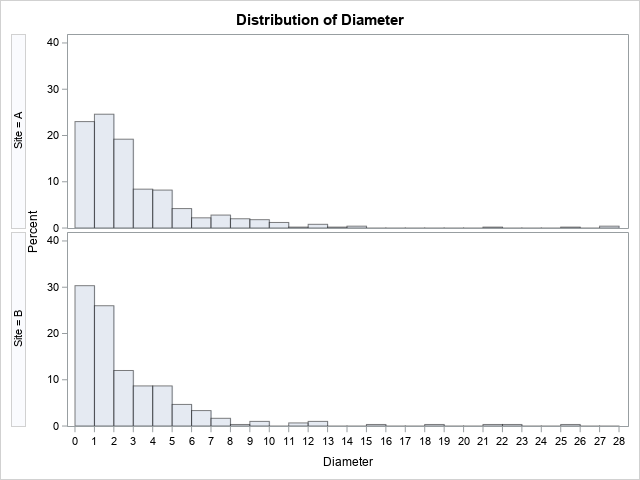For Site 'A', the estimates for the sample median and 84th percentile are 2.05 mm and 5.1 mm, respectively. For Site 'B', the estimates are 1.60 mm and 4.7 mm, respectively. These estimates are close to their respective parameter values.

### Estimate difference in percentiles

The QUANTREG procedure in SAS makes it easy to estimate the difference in the 50th and 84th percentiles between the two groups. The syntax for the QUANTREG procedure is similar to other SAS regression procedures such as PROC GLM:

```proc quantreg data=Grains; class Site; model Diameter = Site / quantile=0.5 0.84; estimate 'Diff in Pctl' Site 1 -1 / CL; run;```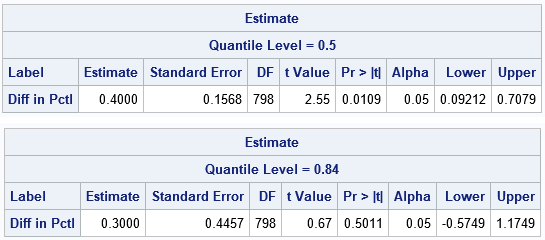The output from PROC QUANTREG shows estimates for the difference between the percentiles of the two groups. For the 50th percentile, an estimate for the difference is 0.4 with a 95% confidence of [0.09, 0.71]. Because 0 is not in the confidence interval, you can conclude that the median pebble size at Site 'A' is significantly different from the median pebble size at Site 'B'. For the 84th percentile, an estimate for the difference is 0.3 with a 95% confidence of [-0.57, 1.17]. Because 0 is in the interval, the difference between the 84th percentiles is not significantly different from 0.

### Methods for estimating confidence intervals

The QUANTREG procedure supports several different methods for estimating a confidence interval: sparsity, rank, and resampling. The estimates in the previous section are by using the RANK method, which is the default for smaller data sets. You can use the CI= option on the PROC QUANTREG statement to use these methods and to specify options for the methods. The following graph summarizes the results for four combinations of methods and options. The results of the analysis do not change: The medians are significantly different but the 84th percentiles are not.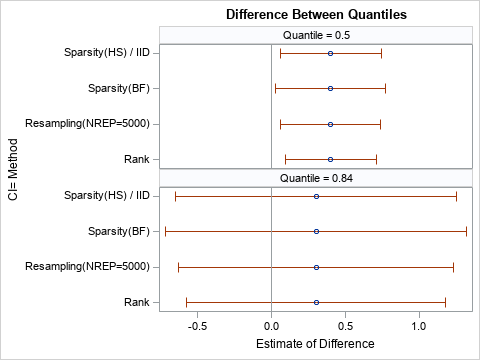### A comparison with bootstrap estimates

When you use a resampling-based estimate for the confidence interval, the interval depends on the random number seed, the algorithm used to generate random numbers, and the number of bootstrap iterations. This can make it hard to compare Monte Carlo results to the results from deterministic statistical methods. Nevertheless, I will present one result from a bootstrap analysis of the simulated data. The following table shows the bootstrap percentile estimates (used by Eaton, Moore, and MacKenzie (2019)) for the difference between percentiles.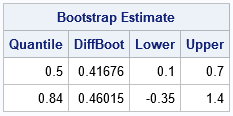The confidence intervals (the Lower and Upper columns of the table) are comparable to the intervals produced by PROC QUANTREG. The confidence interval for the difference between medians seems equivalent. The bootstrap interval for the 84th percentile is shifted to the right relative to the QUANTREG intervals. However, the inferences are the same: the medians are different but there is no significant difference between the 84th percentiles.

The following histogram shows the difference between the 84th percentiles for 5,000 bootstrap samples. The confidence interval is determined by the 2.5th and 97.5th percentiles of the bootstrap distribution. The computation requires only a short SAS/IML program, which runs very quickly.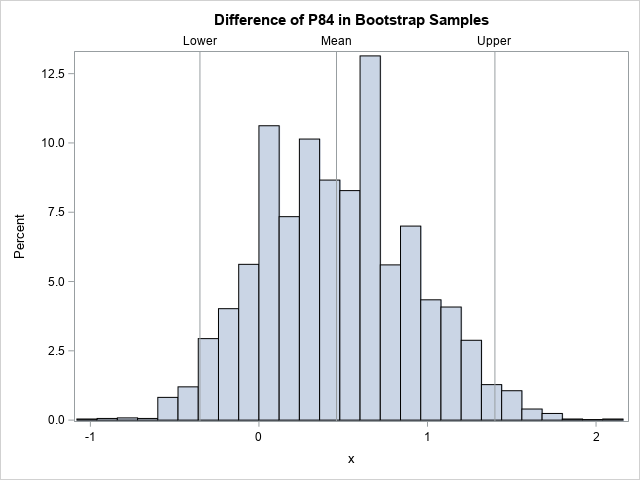### Summary

Data analysts might struggle to find an easy way to compute the difference between percentiles for two (or more) groups. A recent paper by Eaton, Moore, and MacKenzie (2019) proposes one solution, which is to use resampling methods. An alternative is to use quantile regression to estimate the difference between percentiles, as shown in this article. I demonstrate the method by using simulated data of the form studied by Eaton, Moore, and MacKenzie.

The post How to estimate the difference between percentiles appeared first on The DO Loop.In natural language processing, word vectors play a key role in making technologies such as machine translation and speech recognition possible. A word vector is a row of numeric values where each point captures a dimension of the word’s meaning. Each value represents how closely it relates to the concept behind that dimension, so the semantics of the word is embedded across the dimensions of the vector. Since similar words have similar vectors, representing words as vectors like this would simplify and unify vectors' operations.

Word vectors are generated by a training performed word-word co-occurrence statistics on a large corpus. You can use pre-trained word vectors like GloVe, provided by Stanford University.

Let's talk about how to transform word vector tables from long to wide in SAS, so we can potentially get sentence vectors to process further. Suppose we generate word vectors from the following 3 sentences:

Jack went outside.
Jill likes to draw in the afternoon.
Tony is a boy.

Each word has 2 numeric values (Vector1, Vector2), each value represents how closely the word relates to the concept defined by that dimension. The value numbers (here VNUM=2) may range from hundreds to thousands in real text analysis scenarios.

The sample code below generates an upper sample table and sorts it for further processing.

```data HAVE; length Word \$ 45; input SentenceID Word Vector1-Vector2; /*300+*/ datalines; 1 Jacky 0.24011 0.400996 1 went -0.047581 0.868716 1 outside -1.197891 1.162238 2 Jill -0.199579 0.251252 2 likes -1.935640 -0.288264 2 to -0.526053 -1.143420 2 draw -0.736289 -0.794812 2 in -2.757234 0.506639 2 the -0.736289 -0.794812 2 afternoon -0.047581 0.868716 3 Tony 0.34032 0.600983 3 is 0.147531 0.968817 3 a 1.347543 2.568323 3 boy -3.257891 3.172238 run; proc sort data=HAVE; by SentenceID; run; proc print data=have;run;```

If we want to transform the upper long table to a wide table as seen below, how can we do this as efficiently and simply as possible? The upper 14 words belong to 3 sentences that would result in the following 3 rows with 22 columns (1 + WNUM + WNUM x VNUM=1 + 7 + 7 x 2 = 22).

Please note that we can calculate the max word number (WNUM) in a sentence at runtime with SAS code below. For the upper case, the value of WNUM is 7.

```proc sql noprint; select max(count) into :wnum from ( select count(Word) as count from HAVE group by SentenceID ); quit;```

In fact, we don’t need any SAS PROC to handle this kind of transformation. A SAS Data step provides an efficient and convenient way to transform data. The key is to use an ARRAY to map all word vectors from the source table, and then define two ARRAYs to store output words and vectors in a wide style. These two arrays for output words and vectors need to be RETAIN during the implicit loop and KEEP for OUTPUT while it reaches the last SentenceId.

You can see the full SAS code below with detailed comments.

```/*Long table to Wide table*/ %let vnum=2; /*vector numbers for a word*/ %let wnum=7; /*max word number in a sentence*/ data WANT; set HAVE; by Sentenceid; array _vector_ [*] vector:; /*Map to source vectors*/   array _word [ %eval(1*&wnum)] \$ 45; /*Array to store WORD in wide table*/ array _vector [ %eval(&wnum*&vnum)];/*Array to store VECTORS in wide table*/ retain _word: _vector:; /*RETAIN during the implicit loop*/   retain _offset_ 0; /*Offset of a WORD in a sentence, base 0*/ if first.Sentenceid then do; call missing(of _word[*]); call missing(of _vector[*]); _offset_=0; end; else _offset_=_offset_+1;   _word[ _offset_+1 ]=word; /*Cache current word to array WORD at [ _offset_+1]*/ do i=1 to dim(_vector_); /*Cache each vectors to array VECTORS at [_offset_* &vnum +i]*/ _vector[_offset_* &vnum +i]=_vector_[i]; end; keep Sentenceid _word: _vector: ; /*Keep for output when it hit last.Sentenceid*/   if last.Sentenceid then output; /*Output the cached WORD and VECTORS*/ run;   proc print data=want;run;```

Accordingly, if we need to transform a word vector back from wide style to long style, we need to generate &WNUM rows x &VNUM columns for each sentence, and it’s the reversed process for upper logic. The full SAS code with detailed comments is listed below:

```/*Wide table to Long table*/ data HAVE2; set WANT;   array _word [*] _word:; /*Array _word[] mapping to WORD in wide table*/ array _vector_ [*] _vector:; /*Array _vector[] mapping to VECTORS in wide table*/   length Word \$ 45; /*Output Word in the long table*/ array Vector[&vnum]; /*Output Vectors in the long table*/ do i=1 to &wnum; /*Unpack word from array _word[]*/ word=_word[i]; if word=" " then continue; do j=1 to &vnum; /*Unpack vectors from array _vector[]*/ oo= (j+&vnum * (i-1)); Vector[j]=_vector_[j + &vnum *(i-1)]; end; keep Sentenceid Word Vector:; output; /*One row in wide table generate &wnum rows[]*/ end; run; proc print data=HAVE2;run;```

To wrap the upper bi-directional transformation process for general repurposing in text analysis, we provide two SAS MACROs listed below:

```%Long2Wide(data=Have, vnum=2, wnum=7, sid=SentenceId, word=Word, out=Want); proc print data=Want;run;   %Wide2Long(data=Want, vnum=2, wnum=7, sid=Sentenceid, out=Have2, outword=Word, outvector=Vector); proc print data=Have2;run;```

We have demonstrated how to transform a word vector table from a long style to a wide style (or vice versa) efficiently with a SAS DATA step. We have also provided two well-wrapped SAS MACROs for general re-use purposes. To learn more, please check out these additional resources:

Transform word vector tables from long to wide was published on SAS Users.“He spends a lot of time wandering around in circles in the backyard,” my wife said to someone on the telephone. That’s true. Our backyard is only about 1/8th of an acre and I have taken to wandering outside and walking around the fence line. Ostensibly, I am checking to [...]

Data for good: the home edition was published on SAS Voices by Elliot InmanIn a previous article, I discussed the definition of the Kullback-Leibler (K-L) divergence between two discrete probability distributions. For completeness, this article shows how to compute the Kullback-Leibler divergence between two continuous distributions. When f and g are discrete distributions, the K-L divergence is the sum of f(x)*log(f(x)/g(x)) over all x values for which f(x) > 0. When f and g are continuous distributions, the sum becomes an integral:
KL(f,g) = ∫ f(x)*log( f(x)/g(x) ) dx
which is equivalent to
KL(f,g) = ∫ f(x)*( log(f(x)) – log(g(x)) ) dx
The integral is over the support of f, and clearly g must be positive inside the support of f for the integral to be defined.

### The Kullback-Leibler divergence between normal distributions

I like to perform numerical integration in SAS by using the QUAD subroutine in the SAS/IML language. You specify the function that you want to integrate (the integrand) and the domain of integration and get back the integral on the domain. Use the special missing value .M to indicate "minus infinity" and the missing value .P to indicate "positive infinity."

As a first example, suppose the f(x) is the pdf of the normal distribution N(μ1, σ1) and g(x) is the pdf of the normal distribution N(μ2, σ2). From the definition, you can exactly calculate the Kullback-Leibler divergence between normal distributions:
KL between Normals: log(σ2/σ1) + (σ12 – σ22 + (μ1 – μ2)2) / (2*σ22)

You can define the integrand by using the PDF and LOGPDF functions in SAS. The following computation integrates the distributions on the infinite integral (-∞, ∞), although a "six sigma" interval about μ1, such as [-6, 6], would give the same result:

```proc iml; /* Example 1: K-L divergence between two normal distributions */ start KLDivNormal(x) global(mu1, mu2, sigma1, sigma2); return pdf("Normal", x, mu1, sigma1) # (logpdf("Normal", x, mu1, sigma1) - logpdf("Normal", x, mu2, sigma2)); finish;   /* f is PDF for N(0,1) and g is PDF for N(2,3) */ mu1 = 0; sigma1 = 1; mu2 = 2; sigma2 = 3;   call quad(KL, "KLDivNormal", {.M .P}); /* integral on (-infinity, infinity) */ KLExact = log(sigma2/sigma1) + (sigma1##2 - sigma2##2+ (mu1-mu2)##2) / (2*sigma2##2); print KL KLExact (KL-KLExact)[L="Difference"];```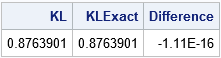The output indicates that the numerical integration agrees with the exact result to many decimal places.

### The Kullback-Leibler divergence between exponential and gamma distributions

A blog post by John D. Cook computes the K-L divergence between an exponential and a gamma(a=2) distribution. This section performs the same computation in SAS.

This is a good time to acknowledge that numerical integration can be challenging. Numerical integration routines have default settings that enable you to integrate a wide variety of functions, but sometimes you need to modify the default parameters to get a satisfactory result—especially for integration on infinite intervals. I have previously written about how to use the PEAK= option for the QUAD routine to achieve convergence in certain cases by providing additional guidance to the QUAD routine about the location and scale of the integrand. Following the advice I gave in the previous article, a value of PEAK=0.1 seems to be a good choice for the K-L divergence between the exponential and gamma(a=2) distributions: it is inside the domain of integration and is close to the mode of the integrand, which is at x=0.

```/* Example 2: K-L divergence between exponential and gamma(2). Example from https://www.johndcook.com/blog/2017/11/08/why-is-kullback-leibler-divergence-not-a-distance/ */ start KLDivExpGamma(x) global(a); return pdf("Exponential", x) # (logpdf("Exponential", x) - logpdf("Gamma", x, a)); finish;   a = 2; /* Use PEAK= option: https://blogs.sas.com/content/iml/2014/08/13/peak-option-in-quad.html */ call quad(KL2, "KLDivExpGamma", {0 .P}) peak=0.1; print KL2;```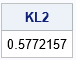The value of the K-L divergence agrees with the answer in John D. Cook's blog post.

### Approximating the Exponential Distribution by the Gamma Distribution

Recall that the K-L divergence is a measure of the dissimilarity between two distributions. For example, the previous example indicates how well the gamma(a=2) distribution approximates the exponential distribution. As I showed for discrete distributions, you can use the K-L divergence to select the parameters for a distribution that make it the most similar to another distribution. You can do this by using optimization methods, but I will merely plot the K-L divergence as a function of the shape parameter (a) to indicate how the K-L divergence depends on the parameter.

You might recall that the gamma distribution includes the exponential distribution as a special case. When the shape parameter a=1, the gamma(1) distribution is an exponential distribution. Therefore, the K-L divergence between the exponential and the gamma(1) distribution should be zero.

The following program computes the K-L divergence for values of a in the range [0.5, 2] and plots the K-L divergence versus the parameter:

```/* Plot KL(Expo, Gamma(a)) for various values of a. Note that gamma(a=1) is the exponential distribution. */ aa = do(0.5, 2, 0.01); KL = j(1, ncol(aa), .); do i = 1 to ncol(aa); a = aa[i]; call quad(KL_i, "KLDivExpGamma", {0 .P}) peak=0.1; KL[i] = KL_i; end; title "Kullback-Leibler Divergence Between Exponential and Gamma{a)"; call series(aa, KL) grid={x y} label={'Gamma Shape Parameter (a)' 'K-L Divergence'};```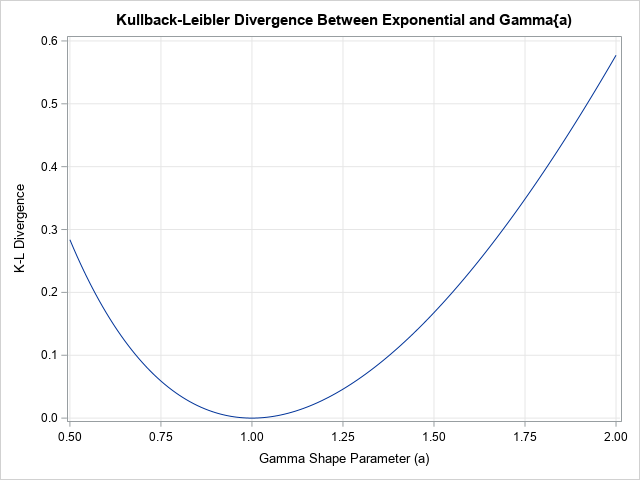As expected, the graph of the K-L divergence reaches a minimum value at a=1, which is the best approximation to an exponential distribution by the gamma(a) distribution. Note that the K-L divergence equals zero when a=1, which indicates that the distributions are identical when a=1.

### The Kullback-Leibler divergence between two continuous probability distributions is an integral. This article shows how to use the QUAD function in SAS/IML to compute the K-L divergence between two probability distributions.

The post The Kullback–Leibler divergence between continuous probability distributions appeared first on The DO Loop.While working at the Rutgers Robert Wood Johnson Medical School, I had access to data on over ten million visits to emergency departments in central New Jersey, including ICD-9 (International Classification of Disease – 9th edition) codes along with some patient demographic data.

I also had the ozone level from several central New Jersey monitoring stations for every hour of the day for ten years. I used PROC REG (and ARIMA) to assess the association between ozone levels and the number of admissions to emergency departments diagnosed as asthma. Some of the predictor variables, besides ozone level, were pollen levels and a dichotomous variable indicating if the date fell on a weekend. (On weekdays, patients were more likely to visit the personal physician than on a weekend.) The study showed a significant association between ozone levels and asthma attacks.

It would have been nice to have the incredible diagnostics that are now produced when you run PROC REG. Imagine if I had SAS Studio back then!

In the program, I used a really interesting trick. (Thank you Paul Grant for showing me this trick so many years ago at a Boston Area SAS User Group meeting.) Here's the problem: there are many possible codes such as 493, 493.9, 493.100, 493.02, and so on that all relate to asthma. The straightforward way to check an ICD-9 code would be to use the SUBSTR function to pick off the first three digits of the code. But why be straightforward when you can be tricky or clever? (Remember Art Carpenter's advice to write clever code that no one can understand so they can't fire you!)

The following program demonstrates the =: operator:

```*An interesting trick to read ICD codes;
<strong>Data</strong> ICD_9;
input ICD : \$7. @@;
if ICD =: "493" the output;
datalines;
493 770.6 999 493.9 493.90 493.100
;
title "Listing of All Asthma Codes";
<strong>proc</strong> <strong>print</strong> data=ICD_9 noobs;
<strong>run</strong>;```

Normally, when SAS compares two strings of different length, it pads the shorter string with blanks to match the length of the longer string before making the comparison. The =: operator truncates the longer string to the length of the shorter string before making the comparison.The usual reason to write a SAS blog is to teach some aspect of SAS programming or to just point out something interesting about SAS. While that is usually my motivation, I have an ulterior motive in writing this blog – I want to plug a new book I have just published on Amazon. It's called 10-8 Awaiting Crew: Memories of a Volunteer EMT. One of the chapters discusses the difficulty of conducting statistical studies in pre-hospital settings. This was my first attempt at a non-technical book. I hope you take a look. (Enter "10-8 awaiting crew" or "Ron Cody" in Amazon search to find the book.) Drop me an email with your thoughts at ron.cody@gmail.com.While working at the Rutgers Robert Wood Johnson Medical School, I had access to data on over ten million visits to emergency departments in central New Jersey, including ICD-9 (International Classification of Disease – 9th edition) codes along with some patient demographic data.

I also had the ozone level from several central New Jersey monitoring stations for every hour of the day for ten years. I used PROC REG (and ARIMA) to assess the association between ozone levels and the number of admissions to emergency departments diagnosed as asthma. Some of the predictor variables, besides ozone level, were pollen levels and a dichotomous variable indicating if the date fell on a weekend. (On weekdays, patients were more likely to visit the personal physician than on a weekend.) The study showed a significant association between ozone levels and asthma attacks.

It would have been nice to have the incredible diagnostics that are now produced when you run PROC REG. Imagine if I had SAS Studio back then!

In the program, I used a really interesting trick. (Thank you Paul Grant for showing me this trick so many years ago at a Boston Area SAS User Group meeting.) Here's the problem: there are many possible codes such as 493, 493.9, 493.100, 493.02, and so on that all relate to asthma. The straightforward way to check an ICD-9 code would be to use the SUBSTR function to pick off the first three digits of the code. But why be straightforward when you can be tricky or clever? (Remember Art Carpenter's advice to write clever code that no one can understand so they can't fire you!)

The following program demonstrates the =: operator:

```*An interesting trick to read ICD codes;
<strong>Data</strong> ICD_9;
input ICD : \$7. @@;
if ICD =: "493" the output;
datalines;
493 770.6 999 493.9 493.90 493.100
;
title "Listing of All Asthma Codes";
<strong>proc</strong> <strong>print</strong> data=ICD_9 noobs;
<strong>run</strong>;```

Normally, when SAS compares two strings of different length, it pads the shorter string with blanks to match the length of the longer string before making the comparison. The =: operator truncates the longer string to the length of the shorter string before making the comparison.The usual reason to write a SAS blog is to teach some aspect of SAS programming or to just point out something interesting about SAS. While that is usually my motivation, I have an ulterior motive in writing this blog – I want to plug a new book I have just published on Amazon. It's called 10-8 Awaiting Crew: Memories of a Volunteer EMT. One of the chapters discusses the difficulty of conducting statistical studies in pre-hospital settings. This was my first attempt at a non-technical book. I hope you take a look. (Enter "10-8 awaiting crew" or "Ron Cody" in Amazon search to find the book.) Drop me an email with your thoughts at ron.cody@gmail.com.The COVID-19 pandemic challenged agriculture and supply chains, but the overarching resilience of agriculture around the world speaks to the industry's efficiency, built-in redundancy and indispensability. In the US, flourishing interactions between government, industry and academic stakeholders underscore how ag represents unity and consilience. And there may be no better [...]

From 9 cows to the future of agtech was published on SAS Voices by John GottulaThe Kullback–Leibler divergence is a measure of dissimilarity between two probability distributions. An application in machine learning is to measure how distributions in a parametric family differ from a data distribution. This article shows that if you minimize the Kullback–Leibler divergence over a set of parameters, you can find a distribution that is similar to the data distribution. This article focuses on discrete distributions.

### The Kullback–Leibler divergence between two discrete distributions

As explained in a previous article, the Kullback–Leibler (K-L) divergence between two discrete probability distributions is the sum
KL(f, g) = Σx f(x) log( f(x)/g(x) )
where the sum is over the set of x values for which f(x) > 0. (The set {x | f(x) > 0} is called the support of f.) For this sum to be well defined, the distribution g must be strictly positive on the support of f.

One application of the K-L divergence is to measure the similarity between a hypothetical model distribution defined by g and an empirical distribution defined by f.

### Example data for the Kullback–Leibler divergence

As an example, suppose a call center averages about 10 calls per hour. An analyst wants to investigate whether the number of calls per hour can be modeled by using a Poisson(λ=10) distribution. To test the hypothesis, the analyst records the number of calls for each hour during 100 hours of operation. The following SAS DATA step reads the data. The call to PROC SGPLOT creates a histogram shows the distribution of the 100 counts:

```data Calls; input N @@; label N = "Calls per hour"; datalines; 11 19 11 13 13 8 11 9 9 14 10 13 8 15 7 9 6 12 7 13 12 19 6 12 11 12 11 9 15 4 7 12 12 10 10 16 18 13 13 8 13 10 9 9 12 13 12 8 13 9 7 9 10 9 4 10 12 5 4 12 8 12 14 16 11 7 18 8 10 13 12 5 11 12 16 9 11 8 11 7 11 15 8 7 12 16 9 18 9 8 10 7 11 12 13 15 6 10 10 7 ;   title "Number of Calls per Hour"; title2 "Data for 100 Hours"; proc sgplot data=Calls; histogram N / scale=proportion binwidth=1; xaxis values=(4 to 19) valueshint; run;```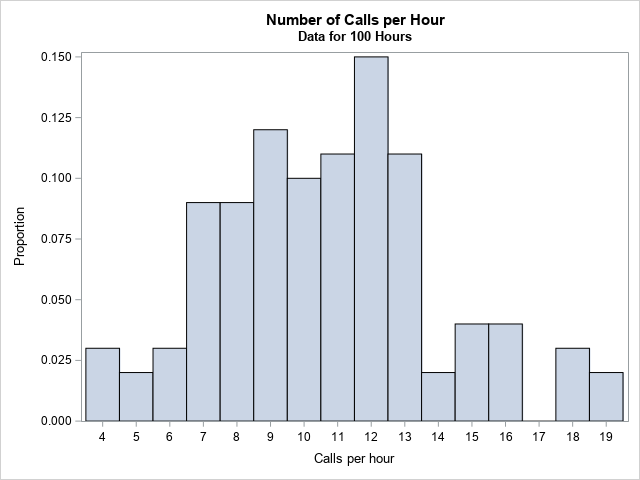The graph should really be a bar chart, but I used a histogram with BINWIDTH=1 so that the graph reveals that the value 17 does not appear in the data. Furthermore, the values 0, 1, 2, and 3 do not appear in the data. I used the SCALE=PROPORTION option to plot the data distribution on the density scale.

The call center wants to model these data by using a Poisson distribution. The traditional statistical approach is to use maximum likelihood estimation (MLE) to find the parameter, λ, in the Poisson family so that the Poisson(λ) distribution is the best fit to the data. However, let's see how using the Kullback–Leibler divergence leads to a similar result.

### The Kullback–Leibler divergence between data and a Poisson distribution

Let's compute the K-L divergence between the empirical frequency distribution and a Poisson(10) distribution. The empirical distribution is the reference distribution; the Poisson(10) distribution is the model. The Poisson distribution has a nonzero probability for all x ≥ 0, but recall that the K-L divergence is computed by summing over the observed values of the empirical distribution, which is the set {4, 5, ..., 19}, excluding the value 17.

```proc iml; /* read the data, which is the reference distribution, f */ use Calls; read all var "N" into Obs; close; call Tabulate(Levels, Freq, Obs); /* find unique values and frequencies */ Proportion = Freq / nrow(Obs); /* empirical density of frequency of calls (f) */   /* create the model distribution: Poisson(10) */ lambda = 10; poisPDF = pdf("Poisson", Levels, lambda); /* Poisson model on support(f) */   /* load K-L divergence module or include the definition from: https://blogs.sas.com/content/iml/2020/05/26/kullback-leibler-divergence-discrete.html */ load module=KLDiv; KL = KLDiv(Proportion, poisPDF); print KL[format=best5.];```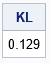Notice that although the Poisson distribution has infinite support, you only need to evaluate the Poisson density on the (finite) support of empirical density.

### Minimize the Kullback–Leibler divergence between data and a Poisson distribution

The previous section shows how to compute the Kullback–Leibler divergence between an empirical density and a Poisson(10) distribution. You can repeat that computation for a whole range of λ values and plot the divergence versus the Poisson parameter. The following statements compute the K-L divergence for λ on [4, 16] and plots the result. The minimum value of the K-L divergence is achieved near λ = 10.7. At that value of λ, the K-L divergence between the data and the Poisson(10.7) distribution is 0.105.

```/* Plot the K-L div versus lambda for a sequence of Poisson(lambda) models */ lambda = do(4, 16, 0.1); KL = j(1, ncol(lambda), .); do i = 1 to ncol(lambda); poisPDF = pdf("Poisson", Levels, lambda[i]); KL[i] = KLDiv(Proportion, poisPDF); end;   title "K-L Divergence from Poisson(lambda)"; call series(lambda, KL) grid={x y} xvalues=4:16 label={'x' 'K-L Divergence'};```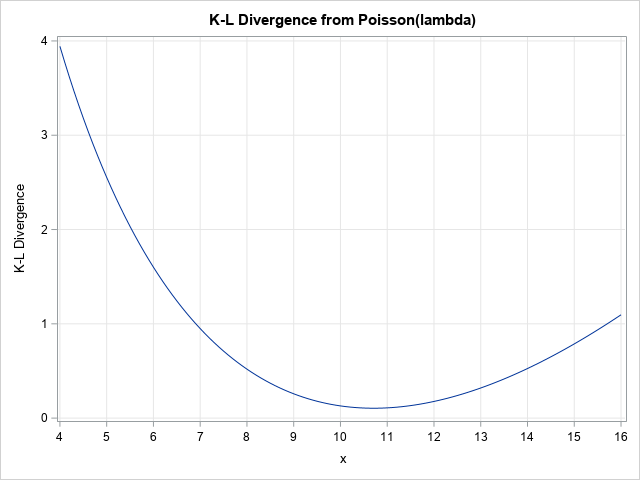The graph shows the K-L divergence for a sequence of Poisson(λ) models. The Poisson(10.7) model has the smallest divergence from the data distribution, therefore it is the most similar to the data among the Poisson(λ) distributions that were considered. You can use a numerical optimization technique in SAS/IML if you want to find a more accurate value that minimizes the K-L divergences.

The following graph overlays the PMF for the Poisson(10.7) distribution on the empirical distribution for the number of calls.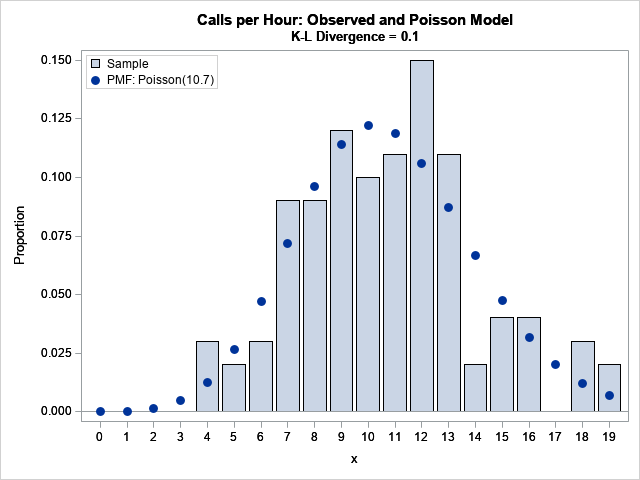### The minimal Kullback–Leibler divergence and the maximum likelihood estimate

You might wonder how minimizing the K-L divergence relates to the traditional MLE method for fitting a Poisson model to the data. The following call to PROC GENMOD shows that the MLE estimate is λ = 10.71:

```proc genmod data=MyData; model Obs = / dist=poisson; output out=PoissonFit p=lambda; run;   proc print data=PoissonFit(obs=1) noobs; var lambda; run;```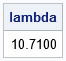Is this a coincidence? No. It turns out that there a connection between the K-L divergence and the negative log-likelihood. Minimizing the K-L divergence is equivalent to minimizing the negative log-likelihood, which is equivalent to maximizing the likelihood between the Poisson model and the data.

### Summary

This article shows how to compute the Kullback–Leibler divergence between an empirical distribution and a Poisson distribution. The empirical distribution was the observed number of calls per hour for 100 hours in a call center. You can compute the K-L divergence for many parameter values (or use numerical optimization) to find the parameter that minimizes the K-L divergence. This parameter value corresponds to the Poisson distribution that is most similar to the data. It turns out that minimizing the K-L divergence is equivalent to maximizing the likelihood function. Although the parameter estimates are the same, the traditional MLE estimate comes with additional tools for statistical inference, such as estimates for confidence intervals and standard errors.

The post Minimizing the Kullback–Leibler divergence appeared first on The DO Loop.